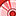# App Development

Discussion board where members can learn more about Qlik Sense App Development and Usage.

Announcements
WE ARE LISTENING! New Navigation for Qlik Community, Sept. 26: TELL ME MORE
cancel
Showing results for
Did you mean:Contributor II

## How to sum against unique ID in set expression

Hi

In my data model, Order ID will repeat multiple time because 1 Order_ID may have more than 1 Item_Line. So all attributes associated with Order_ID are also repeating.

I want to do set analysis as below for Order Amounts but I also want it to count amount once per each unique Order_ID

Sum({ <[Order_Status]={'Pending'},[Order_Date] = {\$(=Year(Today()))}, [Order_Date] = {\$(=Year(Today()+1))}, [Order_Type]={'Online Services'}>} [Order Amount])

how to best write the above set analysis? so it will not double count?

Thanks

Labels (2)

• ### Set Analysis

1 Solution

Accepted SolutionsContributor II
Author

I found the correct Expression

Sum(DISTINCT{ <[Order_Status]={'Pending'},[Order_Date] = {\$(=Year(Today()))}, [Order_Date] = {\$(=Year(Today()+1))}, [Order_Type]={'Online Services'}>}AGGR ([Order Amount],Order_ID))

Thank you everyone

3 RepliesSpecialist III

Would that be the same as Count(Distinct Order_ID)Contributor II
Author

NO, it will be different. Count(Distinct Order_ID) will only count unique order IDs

What I want is some of the order amount but i dont want it to recount the order amount twice if the Order ID row is repeating.Contributor II
Author

I found the correct Expression

Sum(DISTINCT{ <[Order_Status]={'Pending'},[Order_Date] = {\$(=Year(Today()))}, [Order_Date] = {\$(=Year(Today()+1))}, [Order_Type]={'Online Services'}>}AGGR ([Order Amount],Order_ID))

Thank you everyone